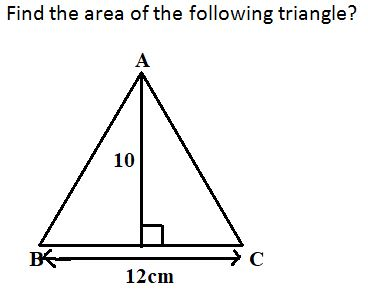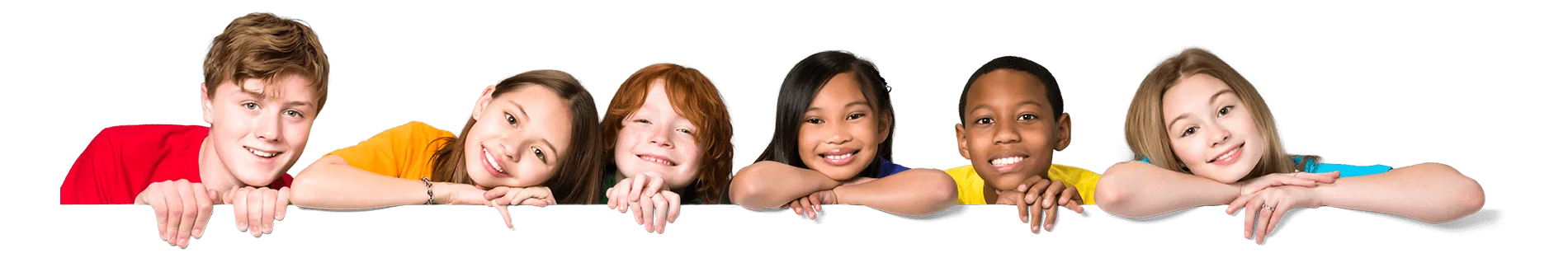Latest Results:

# 11plus Triangle sample papers

Solve this Question

#### Find the angle B in the below triangle?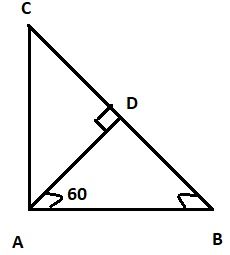1. 60° 2. 40° 3. 30° 4. 20°

Solve this Question

#### A rod of 12 mm is cut into three equal pieces and used to form a triangle. Find the height of the triangle.

 1. 3√3 2. √3 3. 2√3 4. 3

Solve this Question

#### Find the area of the below figure?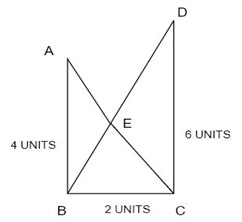1. 10+√3 square units 2. 10-√3 square units 3. 5-√3 square units 4. 5+√3 square units

Solve this Question

#### Two right-angled triangles are combined to form a new triangle as shown below?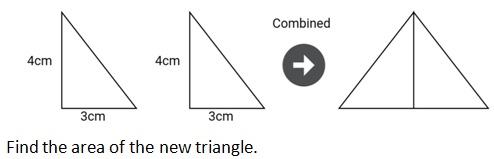1. 22 sq.cm 2. 24 sq.cm 3. 12 sq.cm 4. 6 sq.cm

####